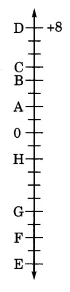Q

# Adjacent figure is a vertical number line, representing integers. Observe it and locate the following points: (a) If point D is + 8, then which point is - 8?

4.   Adjacent figure is a vertical number line, representing integers. Observe it and locate the following points :

(a) If point D is , then which point is ?

(b) Is point G a negative integer or a positive integer?

(c) Write integers for points B and E.

(d) Which point marked on this number line has the least value?

(e) Arrange all the points in decreasing order of value

Views(a) If D is a  then F represents  (by counting on the vertical number line)

(b) G is a negative integer because it is below than point zero (0)

(c) Integers for point B and E are  and  respectively.

(d) E has the least value of

(e) Decreasing order of all the points are-

Exams
Articles
Questions